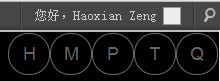# 对 WordPress Lazy Load 图片延迟加载插件稍作改进

2012.09.04

## 1. 将 Lazy Load 效果扩展作用于评论作者头像¶

：0.5 版开始，Lazy Load 已加入对 Avatar 图片的支持。

```// get_avatar filter
if ( ! is_admin() ) // 在 WP 控制台（Dashboard）中的时候不应用此效果
add_filter( 'get_avatar', array( __CLASS__, 'add_image_placeholders' ), 11 );```## 2. 合并 Lazy Load 内部的 2 个 JavaScript 文件¶

• jquery.sonar.js – 未经压缩；仅存档，页面中不使用，与下一文件内容相同
• jquery.sonar.min.js – 经过压缩，页面中调用，实现 Lazy Load 效果，内容同上
• lazy-load.js – 调用 jquery.sonar.min.js 中定义的函数来动态处理页面中的内容

1. 新建一个 all.js 文件，放在 Lazy Load 插件文件夹下的 /js 文件夹下；
2. 将 jquery.min.js 中的内容复制进 all.js；
3. 将 lazy-load.js 中的内容复制进 all.js，放在刚才的内容后面（该文件内容未压缩，能压缩一下就更好，文后附上压缩过的文件内容）；
4. 修改 lazy-load.php 文件，将
```static function add_scripts() {
wp_enqueue_script( 'wpcom-lazy-load-images', self::get_url( 'js/lazy-load.js' ), array( 'jquery', 'jquery-sonar' ), self::version, true );
wp_enqueue_script( 'jquery-sonar', self::get_url( 'js/jquery.sonar.min.js' ), array( 'jquery' ), self::version, true );
}```

替换为，

```static function add_scripts() {
// wp_enqueue_script( 'wpcom-lazy-load-images', self::get_url( 'js/lazy-load.js' ), array( 'jquery', 'jquery-sonar' ), self::version, true );
// wp_enqueue_script( 'jquery-sonar', self::get_url( 'js/jquery.sonar.min.js' ), array( 'jquery' ), self::version, true );
// combine and compress to all.js, cnzhx.net
wp_enqueue_script( 'cnzhx-sonar-lazyload', self::get_url( 'js/all.js' ), array( 'jquery' ), self::version, true );
}```
5. 保存并上传 all.js 和 修改后的 lazy-load.php 文件。

```(function(e,h,l,c){e.fn.sonar=function(o,n){if(typeof o==="boolean"){n=o;o=c}return e.sonar(this,o,n)};var f=l.body,a="scrollin",m="scrollout",b=function(r,n,t){if(r){f||(f=l.body);var s=r,u=0,v=f.offsetHeight,o=h.innerHeight||l.documentElement.clientHeight||f.clientHeight||0,q=l.documentElement.scrollTop||h.pageYOffset||f.scrollTop||0,p=r.offsetHeight||0;if(!r.sonarElemTop||r.sonarBodyHeight!==v){if(s.offsetParent){do{u+=s.offsetTop}while(s=s.offsetParent)}r.sonarElemTop=u;r.sonarBodyHeight=v}n=n===c?0:n;return(!(r.sonarElemTop+(t?0:p)<q-n)&&!(r.sonarElemTop+(t?p:0)>q+o+n))}},d={},j=0,i=function(){setTimeout(function(){var s,o,t,q,p,r,n;for(t in d){o=d[t];for(r=0,n=o.length;r<n;r++){q=o[r];s=q.elem;p=b(s,q.px,q.full);if(t===m?!p:p){if(!q.tr){if(s[t]){e(s).trigger(t);q.tr=1}else{o.splice(r,1);r--;n--}}}else{q.tr=0}}}},25)},k=function(n,o){n[o]=0},g=function(r,p){var t=p.px,q=p.full,s=p.evt,o=b(r,t,q),n=0;r[s]=1;if(s===m?!o:o){setTimeout(function(){e(r).trigger(s===m?m:a)},0);n=1}d[s].push({elem:r,px:t,full:q,tr:n});if(!j){e(h).bind("scroll",i);j=1}};e.sonar=b;d[a]=[];e.event.special[a]={add:function(n){var p=n.data||{},o=this;if(!o[a]){g(this,{px:p.distance,full:p.full,evt:a})}},remove:function(n){k(this,a)}};d[m]=[];e.event.special[m]={add:function(n){var p=n.data||{},o=this;if(!o[m]){g(o,{px:p.distance,full:p.full,evt:m})}},remove:function(n){k(this,m)}}})(jQuery,window,document);

## 3 条关于 “对 WordPress Lazy Load 图片延迟加载插件稍作改进” 的评论

1.神爱 留言：

个人博客感觉用处不大…
图片什么的很少…
图片站需要啊…

•Haoxian Zeng 留言：

嗯，这个就看情况用吧。我最近发现有些页面图片太多，一次性全都加载的话带宽吃紧，所以就用上了这个。